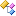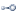﻿VDS.RDF.Query.Algebra Namespace

# VDS.RDF.Query.Algebra Namespace

Contains the classes which model the mapping of SPARQL queries into the SPARQL Algebra. This namespace is a key component of the new Leviathan SPARQL engine introduced in the 0.2.x builds of dotNetRDF.Classes
ClassDescriptionAlgebraExtensions
Static Helper class containing extensions used in the Algebra evaluation process.Ask
Represents the Ask step of Query Evaluation.AskAnyTriples
Special Algebra Construct for optimising queries of the form ASK WHERE {?s ?p ?o}.AskBgp
Represents a BGP which is a set of Triple Patterns.AskUnion
Represents a Union.BaseArbitraryLengthPathOperator
Abstract Base Class for Arbitrary Length Path Operators.BaseMultiset
Abstract Base Class for representing Multisets.BasePathOperator
Abstract Base Class for Path Operators.BaseSet
Abstract Base Class for implementations of the ISet interface.Bgp
Represents a BGP which is a set of Triple Patterns.Bindings
Represents a BINDINGS modifier on a SPARQL Query.Distinct
Represents a Distinct modifier on a SPARQL Query.ExistsJoin
Represents a LeftJoin predicated on the existence/non-existence of joinable sets on the RHS for each item on the LHS.Extend
Represents an Extend operation which is the formal algebraic form of the BIND operation.Filter
Represents a Filter.FilteredProduct
Algebra operator which combines a Filter and a Product into a single operation for improved performance and reduced memory usage.FullTextQuery
Algebra Operator which provides full text query capabilities for a query.Graph
Represents a GRAPH clause.GroupBy
Represents a Grouping.GroupMultiset
Multiset which represents a Grouping of Sets from another Multiset.Having
Represents a Having Clause.IdentityFilter
Represents a special case Filter where the Filter restricts a variable to just one value i.e. FILTER(?x = <value>).IdentityMultiset
Represents the Identity Multiset.Join
Represents a Join.LazyBgp
Represents a BGP which is a set of Triple Patterns.LazyUnion
Represents a Union.LeftJoin
Represents a LeftJoin predicated on an arbitrary filter expression.Minus
Represents the Minus join.Multiset
Represents a Multiset of possible solutions.NegatedPropertySet
Represents a Negated Property Set in the SPARQL Algebra.NullMultiset
Represents a Multiset when there are no possible Solutions.NullOperator
Represents a part of the algebra that has been determined to not return any results in advance and so can be replaced with this operator which always returns null.OneOrMorePath
Represents a One or More Path (e.g. rdf:type+) in the SPARQL Algebra.OrderBy
Represents an Order By clause.ParallelJoin
Represents a Join which will be evaluated in parallel.ParallelUnion
Represents a Union which will be evaluated in parallel.PartitionedMultiset
Implementation of a multiset which is suitable for multiple threads to write to in parallel, useful for parallelizing certain operations.PropertyFunction
Algebra that represents the application of a Property Function.PropertyPath
Represents an arbitrary property path in the algebra (only used when strict algebra is generated).Reduced
Represents a Reduced modifier on a SPARQL Query.SameTermFilter
Represents a special case Filter where the Filter is supposed to restrict a variable to just one value i.e. FILTER(SAMETERM(?x, <value>)).Select
Represents the Selection step of Query Evaluation.SelectDistinctGraphs
Special Algebra Construct for optimising queries of the form SELECT DISTINCT ?g WHERE {GRAPH ?g {?s ?p ?o}}.Service
Represents a Service Clause.Set
Represents one possible set of values which is a solution to the query.SetDistinctnessComparer
Comparer for checking whether sets are distinct, check may either be using the entire set or by using only a subset of variables.SingleValueRestrictionFilter
Abstract Base Class for specialised Filters which restrict the value of a variable to a single value.Slice
Represents the Slice Operation in the SPARQL Algebra.SubQuery
Represents a sub-query as an Algebra operator (only used when strict algebra is generated).Table
Represents a fixed set of solutions.Union
Represents a Union.VariableRestrictionFilter
Abstract Base Class for specialised Filters which restrict the value of a variable to some values.ZeroLengthPath
Represents a Zero Length Path in the SPARQL Algebra.ZeroOrMorePath
Represents a Zero or More Path in the SPARQL Algebra.Interfaces
InterfaceDescriptionIAbstractJoin
Represents an Algebra construct which is an Abstract Join (i.e. any kind of Join over two algebra operators).IBgp
Represents an Algebra construct which is a BGP.IExistsJoin
Represents an Algebra construct which is an Exists Join.IFilter
Represents an Algebra construct which is a Filter.IJoin
Represents an Algebra construct which is a Join.ILeftJoin
Represents an Algebra construct which is a Left Join.IMinus
Represents an Algebra construct which is a Minus.IPathOperator
Interface for Property Path Operators.ISet
Interface for Sets which represents a possible solution during SPARQL evaluation.ISparqlAlgebra
Interface for classes that represent the SPARQL Algebra and are used to evaluate queries.ITerminalOperator
Marker Interface for SPARQL Algebra constructs which are terminal operators i.e. they contain no inner algebra operators.IUnaryOperator
Interface for SPARQL Algebra constructs which are unary operators i.e. they apply over a single inner Algebra.IUnion
Represents an Algebra construct which is a Union.